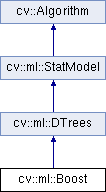OpenCV  3.1.0 Open Source Computer Vision
cv::ml::Boost Class Referenceabstract

Boosted tree classifier derived from DTrees. More...

#include "ml.hpp"

Inheritance diagram for cv::ml::Boost:## Public Types

enum  Types {
DISCRETE =0,
REAL =1,
LOGIT =2,
GENTLE =3
}Public Types inherited from cv::ml::DTrees
enum  Flags {
PREDICT_AUTO =0,
PREDICT_SUM =(1<<8),
PREDICT_MAX_VOTE =(2<<8),
}Public Types inherited from cv::ml::StatModel
enum  Flags {
UPDATE_MODEL = 1,
RAW_OUTPUT =1,
COMPRESSED_INPUT =2,
PREPROCESSED_INPUT =4
}

## Public Member Functions

virtual int getBoostType () const =0

virtual int getWeakCount () const =0

virtual double getWeightTrimRate () const =0

virtual void setBoostType (int val)=0

virtual void setWeakCount (int val)=0

virtual void setWeightTrimRate (double val)=0Public Member Functions inherited from cv::ml::DTrees
virtual int getCVFolds () const =0

virtual int getMaxCategories () const =0

virtual int getMaxDepth () const =0

virtual int getMinSampleCount () const =0

virtual const std::vector< Node > & getNodes () const =0
Returns all the nodes. More...

virtual cv::Mat getPriors () const =0
The array of a priori class probabilities, sorted by the class label value. More...

virtual float getRegressionAccuracy () const =0

virtual const std::vector< int > & getRoots () const =0
Returns indices of root nodes. More...

virtual const std::vector< Split > & getSplits () const =0
Returns all the splits. More...

virtual const std::vector< int > & getSubsets () const =0
Returns all the bitsets for categorical splits. More...

virtual bool getTruncatePrunedTree () const =0

virtual bool getUse1SERule () const =0

virtual bool getUseSurrogates () const =0

virtual void setCVFolds (int val)=0

virtual void setMaxCategories (int val)=0

virtual void setMaxDepth (int val)=0

virtual void setMinSampleCount (int val)=0

virtual void setPriors (const cv::Mat &val)=0
The array of a priori class probabilities, sorted by the class label value. More...

virtual void setRegressionAccuracy (float val)=0

virtual void setTruncatePrunedTree (bool val)=0

virtual void setUse1SERule (bool val)=0

virtual void setUseSurrogates (bool val)=0Public Member Functions inherited from cv::ml::StatModel
virtual float calcError (const Ptr< TrainData > &data, bool test, OutputArray resp) const
Computes error on the training or test dataset. More...

virtual bool empty () const
Returns true if the Algorithm is empty (e.g. in the very beginning or after unsuccessful read. More...

virtual int getVarCount () const =0
Returns the number of variables in training samples. More...

virtual bool isClassifier () const =0
Returns true if the model is classifier. More...

virtual bool isTrained () const =0
Returns true if the model is trained. More...

virtual float predict (InputArray samples, OutputArray results=noArray(), int flags=0) const =0
Predicts response(s) for the provided sample(s) More...

virtual bool train (const Ptr< TrainData > &trainData, int flags=0)
Trains the statistical model. More...

virtual bool train (InputArray samples, int layout, InputArray responses)
Trains the statistical model. More...Public Member Functions inherited from cv::Algorithm
Algorithm ()

virtual ~Algorithm ()

virtual void clear ()
Clears the algorithm state. More...

virtual String getDefaultName () const

virtual void read (const FileNode &fn)
Reads algorithm parameters from a file storage. More...

virtual void save (const String &filename) const

virtual void write (FileStorage &fs) const
Stores algorithm parameters in a file storage. More...

## Static Public Member Functions

static Ptr< Boostcreate ()Static Public Member Functions inherited from cv::ml::DTrees
static Ptr< DTreescreate ()
Creates the empty model. More...Static Public Member Functions inherited from cv::ml::StatModel
template<typename _Tp >
static Ptr< _Tp > train (const Ptr< TrainData > &data, int flags=0)
Create and train model with default parameters. More...Static Public Member Functions inherited from cv::Algorithm
template<typename _Tp >
static Ptr< _Tp > load (const String &filename, const String &objname=String())
Loads algorithm from the file. More...

template<typename _Tp >
static Ptr< _Tp > loadFromString (const String &strModel, const String &objname=String())
Loads algorithm from a String. More...

template<typename _Tp >
static Ptr< _Tp > read (const FileNode &fn)
Reads algorithm from the file node. More...

## Detailed Description

Boosted tree classifier derived from DTrees.

Boosting

## Member Function Documentation

 static Ptr cv::ml::Boost::create ( )
static

Creates the empty model. Use StatModel::train to train the model, Algorithm::load<Boost>(filename) to load the pre-trained model.

 virtual int cv::ml::Boost::getBoostType ( ) const
pure virtual

Type of the boosting algorithm. See Boost::Types. Default value is Boost::REAL.

setBoostType
 virtual int cv::ml::Boost::getWeakCount ( ) const
pure virtual

The number of weak classifiers. Default value is 100.

setWeakCount
 virtual double cv::ml::Boost::getWeightTrimRate ( ) const
pure virtual

A threshold between 0 and 1 used to save computational time. Samples with summary weight $$\leq 1 - weight_trim_rate$$ do not participate in the next iteration of training. Set this parameter to 0 to turn off this functionality. Default value is 0.95.

setWeightTrimRate
 virtual void cv::ml::Boost::setBoostType ( int val )
pure virtual